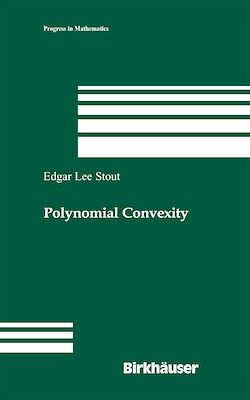439 Pages
English

# Polynomial Convexity

-Description

This book is devoted to an exposition of the theory of polynomially convex sets.Acompact N subset of C is polynomially convex if it is de?ned by a family, ?nite or in?nite, of polynomial inequalities. These sets play an important role in the theory of functions of several complex variables, especially in questions concerning approximation. On the one hand, the present volume is a study of polynomial convexity per se, on the other, it studies the application of polynomial convexity to other parts of complex analysis, especially to approximation theory and the theory of varieties. N Not every compact subset of C is polynomially convex, but associated with an arbitrary compact set, say X, is its polynomially convex hull, X, which is the intersection of all polynomially convex sets that contain X. Of paramount importance in the study of polynomial convexity is the study of the complementary set X \ X. The only obvious reason for this set to be nonempty is for it to have some kind of analytic structure, and initially one wonders whether this set always has complex structure in some sense. It is not long before one is disabused of this naive hope; a natural problem then is that of giving conditions under which the complementary set does have complex structure. In a natural class of one-dimensional examples, such analytic structure is found. The study of this class of examples is one of the major directions of the work at hand.

Subjects

##### Abstract

Informations